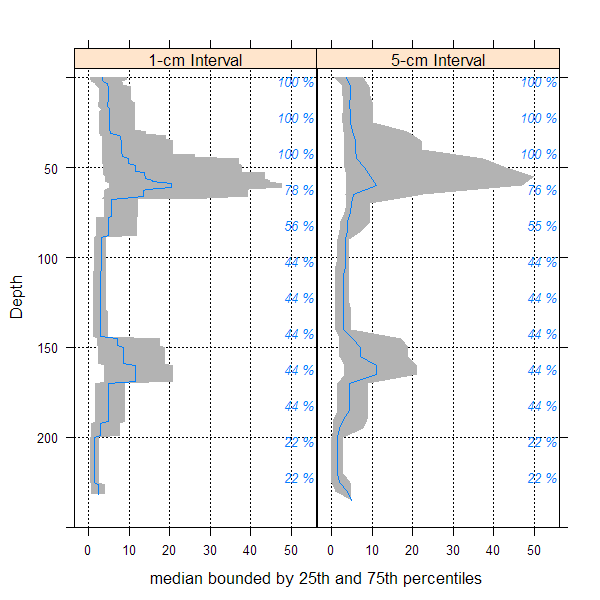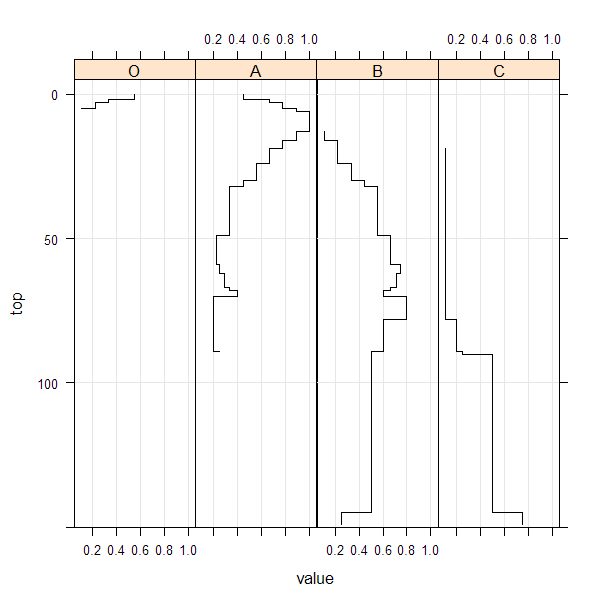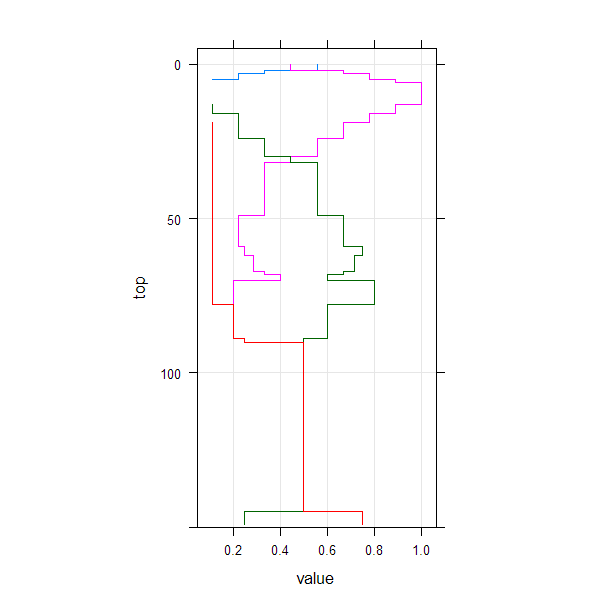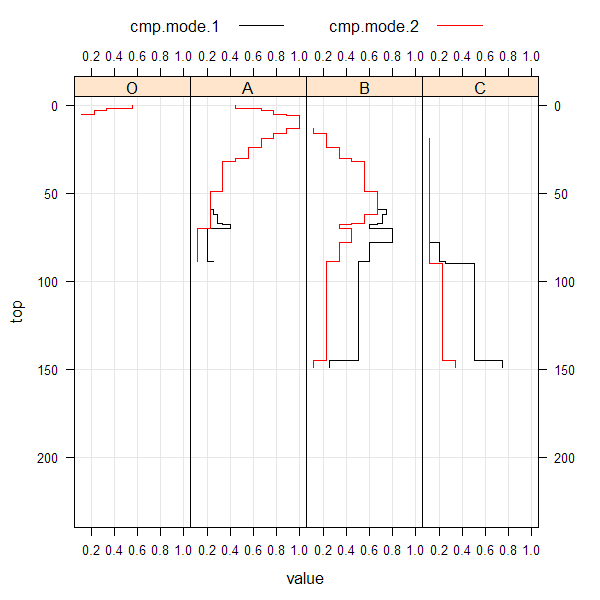slab-methods {aqp} R Documentation

## Slab-Wise Aggregation of SoilProfileCollection Objects

### Description

Aggregate soil properties along user-defined 'slabs', and optionally within groups.

### Usage

```# method for SoilProfileCollection objects
slab(object, fm, slab.structure=1, strict=FALSE,
slab.fun=.slab.fun.numeric.default, cpm=1, weights=NULL, ...)
```

### Arguments

 `object` a SoilProfileCollection `fm` A formula: either ‘groups ~ var1 + var2 + var3’ where named variables are aggregated within ‘groups’ OR where named variables are aggregated across the entire collection ‘ ~ var1 + var2 + var3’. If 'groups' is a factor it must not contain NA. `slab.structure` A user-defined slab thickness (defined by an integer), or user-defined structure (numeric vector). See details below. `strict` logical: should horizons be strictly checked for self-consistency? `slab.fun` Function used to process each 'slab' of data, ideally returning a vector with names attribute. Defaults to a wrapper function around `hdquantile`. See details. `cpm` Strategy for normalizing slice-wise probabilities, dividing by either: number of profiles with data at the current slice (cpm=1), or by the number of profiles in the collection (cpm=2). Mode 1 values will always sum to the contributing fraction, while mode 2 values will always sum to 1. `weights` Column name containing weights. NOT YET IMPLEMENTED `...` further arguments passsed to `slab.fun`

### Details

Multiple continuous variables OR a single categorical (factor) variable can be aggregated within a call to `slab`. Basic error checking is performed to make sure that top and bottom horizon boundaries make sense. User-defined aggregate functions (`slab.fun`) should return a named vector of results. A new, named column will appear in the results of `slab` for every named element of a vector returned by `slab.fun`. See examples below for a simple example of a slab function that computes mean, mean-1SD and mean+1SD. The default slab function wraps `hdquantile` from the Hmisc package, which requires at least 2 observations per chunk. Note that if 'group' is a factor it must not contain NAs.

Execution time scales linearly (slower) with the total number of profiles in `object`, and exponentially (faster) as the number of profiles / group is increased. `slab()` and `slice()` are much faster and require less memory if input data are either numeric or character.

There are several possible ways to define slabs, using `slab.structure`:

a single integer

e.g. 10: data are aggregated over a regular sequence of 10-unit thickness slabs

a vector of 2 integers

e.g. c(50, 60): data are aggregated over depths spanning 50–60 units

a vector of 3 or more integers

e.g. c(0, 5, 10, 50, 100): data are aggregated over the depths spanning 0–5, 5–10, 10–50, 50–100 units

### Value

Output is returned in long format, such that slice-wise aggregates are returned once for each combination of grouping level (optional), variable described in the `fm` argument, and depth-wise 'slab'.

Aggregation of numeric variables, using the default slab function:

variable

The names of variables included in the call to `slab`.

groupname

The name of the grouping variable when provided, otherwise a fake grouping variable named 'all.profiles'.

p.q5

The slice-wise 5th percentile.

p.q25

The slice-wise 25th percentile

p.q50

The slice-wise 50th percentile (median)

p.q75

The slice-wise 75th percentile

p.q95

The slice-wise 95th percentile

top

The slab top boundary.

bottom

The slab bottom boundary.

contributing_fraction

The fraction of profiles contributing to the aggregate value, ranges from 1/n_profiles to 1.

When a single factor variable is used, slice-wise probabilities for each level of that factor are returned as:

variable

The names of variables included in the call to `slab`.

groupname

The name of the grouping variable when provided, otherwise a fake grouping variable named 'all.profiles'.

A

The slice-wise probability of level A

B

The slice-wise probability of level B

...
n

The slice-wise probability of level n

top

The slab top boundary.

bottom

The slab bottom boundary.

contributing_fraction

The fraction of profiles contributing to the aggregate value, ranges from 1/n_profiles to 1.

### Methods

data = "SoilProfileCollection"

Typical usage, where input is a `SoilProfileCollection`.

### Note

Arguments to `slab` have changed with `aqp` 1.5 (2012-12-29) as part of a code clean-up and optimization. Calculation of weighted-summaries was broken in `aqp` 1.2-6 (2012-06-26), and removed as of `aqp` 1.5 (2012-12-29). `slab` replaced the previously defined `soil.slot.multiple` function as of `aqp` 0.98-8.58 (2011-12-21).

D.E. Beaudette

### References

D.E. Beaudette, P. Roudier, A.T. O'Geen, Algorithms for quantitative pedology: A toolkit for soil scientists, Computers & Geosciences, Volume 52, March 2013, Pages 258-268, 10.1016/j.cageo.2012.10.020.

Harrell FE, Davis CE (1982): A new distribution-free quantile estimator. Biometrika 69:635-640.

`slice, hdquantile`

### Examples

``````##
## basic examples
##
library(lattice)
library(grid)

# load sample data, upgrade to SoilProfileCollection
data(sp1)
depths(sp1) <- id ~ top + bottom

# aggregate entire collection with two different segment sizes
a <- slab(sp1, fm = ~ prop)
b <- slab(sp1, fm = ~ prop, slab.structure=5)

# check output
str(a)
``````
``````## 'data.frame':    240 obs. of  10 variables:
##  \$ variable             : Factor w/ 1 level "prop": 1 1 1 1 1 1 1 1 1 1 ...
##  \$ all.profiles         : num  1 1 1 1 1 1 1 1 1 1 ...
##  \$ p.q5                 : num  0.0083 0.0083 0.0332 0.1541 0.1541 ...
##  \$ p.q25                : num  0.474 0.474 0.771 1.552 1.552 ...
##  \$ p.q50                : num  3.44 3.44 3.52 4.53 4.53 ...
##  \$ p.q75                : num  9.52 9.52 8.34 8.51 8.51 ...
##  \$ p.q95                : num  13.7 13.7 16.6 16.6 16.6 ...
##  \$ top                  : int  0 1 2 3 4 5 6 7 8 9 ...
##  \$ bottom               : int  1 2 3 4 5 6 7 8 9 10 ...
##  \$ contributing_fraction: num  1 1 1 1 1 1 1 1 1 1 ...
``````
``````# stack into long format
ab <- make.groups(a, b)
ab\$which <- factor(ab\$which, levels=c('a','b'),
labels=c('1-cm Interval', '5-cm Interval'))

# plot median and IQR
# custom plotting function for uncertainty viz.
xyplot(top ~ p.q50 | which, data=ab, ylab='Depth',
xlab='median bounded by 25th and 75th percentiles',
lower=ab\$p.q25, upper=ab\$p.q75, ylim=c(250,-5),
panel=panel.depth_function,
prepanel=prepanel.depth_function,
cf=ab\$contributing_fraction,
layout=c(2,1), scales=list(x=list(alternating=1))
)
````````````##
## categorical variable example
##
library(reshape)

# normalize horizon names: result is a factor
sp1\$name <- generalize.hz(sp1\$name,
new=c('O','A','B','C'),
pat=c('O', '^A','^B','C'))

# compute slice-wise probability so that it sums to contributing fraction, from 0-150
a <- slab(sp1, fm= ~ name, cpm=1, slab.structure=0:150)

# reshape into long format for plotting
a.long <- melt(a, id.vars=c('top','bottom'), measure.vars=c('O','A','B','C'))

# plot horizon type proportions using panels
xyplot(top ~ value | variable, data=a.long, subset=value > 0,
ylim=c(150, -5), type=c('S','g'), horizontal=TRUE, layout=c(4,1), col=1 )
````````````# again, this time using groups
xyplot(top ~ value, data=a.long, groups=variable, subset=value > 0,
ylim=c(150, -5), type=c('S','g'), horizontal=TRUE, asp=2)
````````````# adjust probability to size of collection, from 0-150
a.1 <- slab(sp1, fm= ~ name, cpm=2, slab.structure=0:150)

# reshape into long format for plotting
a.1.long <- melt(a.1, id.vars=c('top','bottom'), measure.vars=c('O','A','B','C'))

# combine aggregation from `cpm` modes 1 and 2
g <- make.groups(cmp.mode.1=a.long, cmp.mode.2=a.1.long)

# plot horizon type proportions
xyplot(top ~ value | variable, groups=which, data=g, subset=value > 0,
ylim=c(240, -5), type=c('S','g'), horizontal=TRUE, layout=c(4,1),
auto.key=list(lines=TRUE, points=FALSE, columns=2),
par.settings=list(superpose.line=list(col=c(1,2))),
scales=list(alternating=3))
````````````# apply slice-wise evaluation of max probability, and assign ML-horizon at each slice
(gen.hz.ml <- get.ml.hz(a, c('O','A','B','C')))
``````
``````##   hz top bottom confidence pseudo.brier
## 1  O   0      2         37    0.3950617
## 2  A   2     32         75    0.1547325
## 3  B  32    145         57    0.3574667
## 4  C 145    150         71    0.1250000
``````
``````## Not run:
##D ##
##D ## multivariate examples
##D ##
##D data(sp3)
##D
##D # add new grouping factor
##D sp3\$group <- 'group 1'
##D sp3\$group[as.numeric(sp3\$id) > 5] <- 'group 2'
##D sp3\$group <- factor(sp3\$group)
##D
##D # upgrade to SPC
##D depths(sp3) <- id ~ top + bottom
##D site(sp3) <- ~ group
##D
##D # custom 'slab' function, returning mean +/- 1SD
##D mean.and.sd <- function(values) {
##D     m <- mean(values, na.rm=TRUE)
##D     s <- sd(values, na.rm=TRUE)
##D     upper <- m + s
##D     lower <- m - s
##D     res <- c(mean=m, lower=lower, upper=upper)
##D     return(res)
##D     }
##D
##D # aggregate several variables at once, within 'group'
##D a <- slab(sp3, fm=group ~ L + A + B, slab.fun=mean.and.sd)
##D
##D # check the results:
##D # note that 'group' is the column containing group labels
##D library(lattice)
##D xyplot(
##D     top ~ mean | variable, data=a, groups=group,
##D     lower=a\$lower, upper=a\$upper, sync.colors=TRUE, alpha=0.5,
##D     cf=a\$contributing_fraction,
##D     ylim=c(125,-5), layout=c(3,1), scales=list(x=list(relation='free')),
##D     par.settings=list(superpose.line=list(lwd=2, col=c('RoyalBlue', 'Orange2'))),
##D     panel=panel.depth_function,
##D     prepanel=prepanel.depth_function,
##D     auto.key=list(columns=2, lines=TRUE, points=FALSE)
##D )
##D
##D
##D # compare a single profile to the group-level aggregate values
##D a.1 <- slab(sp3[1, ], fm=group ~ L + A + B, slab.fun=mean.and.sd)
##D
##D # manually update the group column
##D a.1\$group <- 'profile 1'
##D
##D # combine into a single data.frame:
##D g <- rbind(a, a.1)
##D
##D # plot with customized line styles
##D xyplot(
##D     top ~ mean | variable, data=g, groups=group, subscripts=TRUE,
##D     lower=a\$lower, upper=a\$upper, ylim=c(125,-5),
##D     layout=c(3,1), scales=list(x=list(relation='free')),
##D     panel=panel.depth_function,
##D     prepanel=prepanel.depth_function,
##D     sync.colors=TRUE, alpha=0.25,
##D     par.settings=list(superpose.line=list(col=c('orange', 'royalblue', 'black'),
##D   lwd=2, lty=c(1,1,2))),
##D     auto.key=list(columns=3, lines=TRUE, points=FALSE)
##D )
##D
##D
##D
##D ## convert mean value for each variable into long format
##D library(reshape)
##D
##D # note that depths are no longer in order
##D a.wide <- cast(a, group + top + bottom ~ variable, value=c('mean'))
##D
##D ## again, this time for a user-defined slab from 40-60 cm
##D a <- slab(sp3, fm=group ~ L + A + B, slab.structure=c(40,60), slab.fun=mean.and.sd)
##D
##D # now we have weighted average properties (within the defined slab)
##D # for each variable, and each group
##D (a.wide <- cast(a, group + top + bottom ~ variable, value=c('mean')))
##D
##D ## this time, compute the weighted mean of selected properties, by profile ID
##D a <- slab(sp3, fm= id ~ L + A + B, slab.structure=c(40,60), slab.fun=mean.and.sd)
##D (a.wide <- cast(a, id + top + bottom ~ variable, value=c('mean')))
##D
##D
##D ## aggregate the entire collection, using default slab function (hdquantile)
##D ## note the missing left-hand side of the formula
##D a <- slab(sp3, fm= ~ L + A + B)
##D
##D
##D ## weighted-aggregation -- NOT YET IMPLEMENTED --
##D # load sample data, upgrade to SoilProfileCollection
##D data(sp1)
##D depths(sp1) <- id ~ top + bottom
##D
##D # generate pretend weights as site-level attribute
##D set.seed(10101)
##D sp1\$site.wts <- runif(n=length(sp1), min=20, max=100)
## End(Not run)
``````

[Package aqp version 1.9.1 Index]